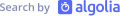## Algolia's latest articles, right in your inbox.

Pick up the topics you are the most interested in, we take care of the rest!

Thank you for subscribing, stay tuned!At one point, every company needs to compute some basic statistics on its data. I’m not speaking about advanced statistics but simple ones, like means, top values, etc. The algorithms to compute those metrics are fairly straightforward, but can take a lot of memory. So what happens when the amount of data is so big that it can’t fit in a reasonable amount of RAM?

Today at Algolia we generate 2 TB of logs per day, so computing metrics on this doesn’t fit into any of our machines. Furthermore, the data team would like to have those metrics in pseudo real time, so we need to process the logs in real time.

# How to compute metrics on terabytes of data

For some of our data, it was OK not to have an exact value but an approximation. For example, instead of having an exact average of 3.9, we get 4.0, and that’s ok. Thankfully, some algorithms and data structures are able to lower their precision to have a lower memory footprint. Those data structures are what we call probabilistic data structures (or algorithms), and they all share some properties:

• Have a small memory footprint — we are talking KB of memory instead of TB.
• Usable in a single pass: each piece of data is processed once by the algorithm.
• No statistical hypothesis on the data being processed.
• Have a precision within an acceptable error margin. Most of the time the margin is a parameter of the algorithm.

We’ll see later how they work.

# How to compute metrics on a stream of events

As we have logs arriving continuously, the team thought that we could leverage this and compute metrics on the fly. There are algorithms designed to process data on the fly, and they are called streaming algorithms. Sure, the name is fancy, but we code them every day. Let’s take an example.

Let’s say we have an array of integers, and we would like to compute the sum of each element. In pseudo code we would probably do something like:

Now imagine that our array is infinite (what we call a stream). Our code won’t change, and at any point in time, the variable sum would contain the sum of all elements we saw. This is a streaming algorithm: an algorithm that can process an infinite number of elements and that is able to give a coherent state after each iteration.

Bonus point: most probabilistic data structures are streaming algorithms.

# How to compute metrics across multiple machines

We are able to compute simple metrics with a small amount of RAM and from a stream of data. But what if your stream is so big that one machine can’t handle it? As we found a way to reduce the memory footprint, couldn’t we find another trick for CPU?

One simple option would be to split the workload across multiple CPUs. For this, we will use a mathematical property. Yes, math. Bear with me a few seconds, you won’t be disappointed.

Let’s say you have a set of elements. On this set you have an operation, let’s call it +, that takes 2 elements of this set and gives back an element of the same set. We say that this set and this operation is a monoid if:

• For any a, b, c that are elements of this set, this holds: (a + b) + c = a + (b + c)
• There exists an element e of this set where: e + a = a + e = a

Let’s take some examples:

• Integers and addition, where e is zero
• Strings and concatenation, where e is the empty string
• Lists and concatenation, where e is the empty list
• Booleans and &&, where e is True

Why did I bother you with this?

Let’s take a simple example, with integers and addition. If you want to sum 1, 2, 3 & 4, you can ask Google or you can spread this sum on multiple CPUs, let’s say 2. Because this is a monoid you know you can do sub additions, for example: 1+2+3+4 = (1+2) + (3+4). So, you can ask the first CPU to compute 1+2, the second one 3+4, and only then sum those sub sums. And voilà, we have our final sum, which is 10.

Therefore, if some set and operation validates 2 properties, we have a sound way to spread the computation on multiple CPUs or machines.

Bonus point: most probabilistic data structures and streaming algorithms are monoids.

# Conclusion

Algorithms like the ones above are not new — far from it. The first probabilistic data structure was invented in 1985 by Philippe Flajolet (hence the pf* commands in redis). Monoids are even older.

Happily for us, a lot of these algorithms are already implemented in big data software (think Spark, Apache Beam, Algebird, etc.).

The main thing we should remember is that some simple mathematical properties give us many nice coding features. Perhaps now you feel sad if you slept through your math classes :).